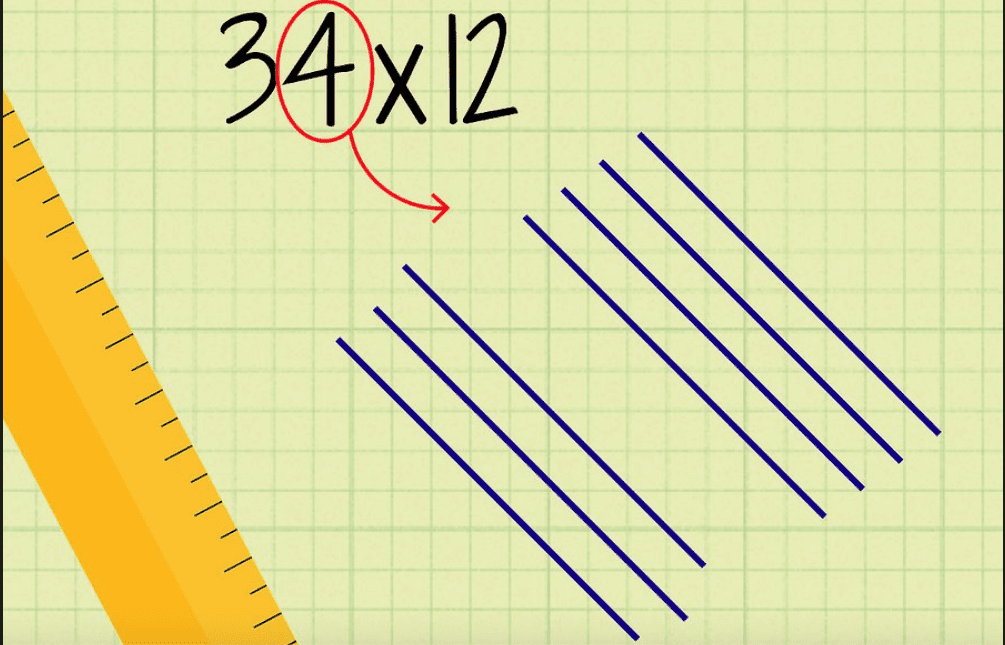# The Line Technique for Multiplication Is Easy and Fun (Actually, Though)

Photo Credit: Wikihow

Since it’s true you do use math every day of your life, you may as well learn a cool way of doing it.

Here’s a method to multiplication that is pretty mind-blowing.

When you multiply numbers on paper, you take the two numbers and stack the larger number on top, lining up the ones column, the tens column and so on. Then you multiply each number on top by the last number (the one in the ones column) on the bottom. You do this with each number on the bottom, then add up your answers for a total.

This is the classic method – the kind I learned in school as a kid and then forgot as an adult.

See? We’re already having a good time.

Let’s try it out by multiplying 5 with 4.

Draw five parallel diagonal lines starting from the lower left up to the upper right. Each line goes to the right of the one before it. Then starting from the upper left, draw four parallel diagonal lines intersecting with your first set. Count the intersections. You will have 20!

Ok, let’s go double digits.

Don’t worry – for this example, we have a series of visual aids.

We’ll calculate 34 multiplied by 12.

Draw three diagonal lines starting from the lower left. Leave some space, then draw four more diagonal lines.Photo Credit: Wikihow

For the 32, draw three diagonal lines from upper left. Leave a space. Draw two more lines. You should have a sort of diamond-shape of empty space in the middle.Photo Credit: Wikihow

Mark the intersections with dots.Photo Credit: Wikihow

Circle all the dots in the right corner – these represent the ones. Then, draw a large circle around the dots in the upper and lower corners – these represent the tens. Finally, circle the dots in the left corner – these represent, you guessed it, the hundreds.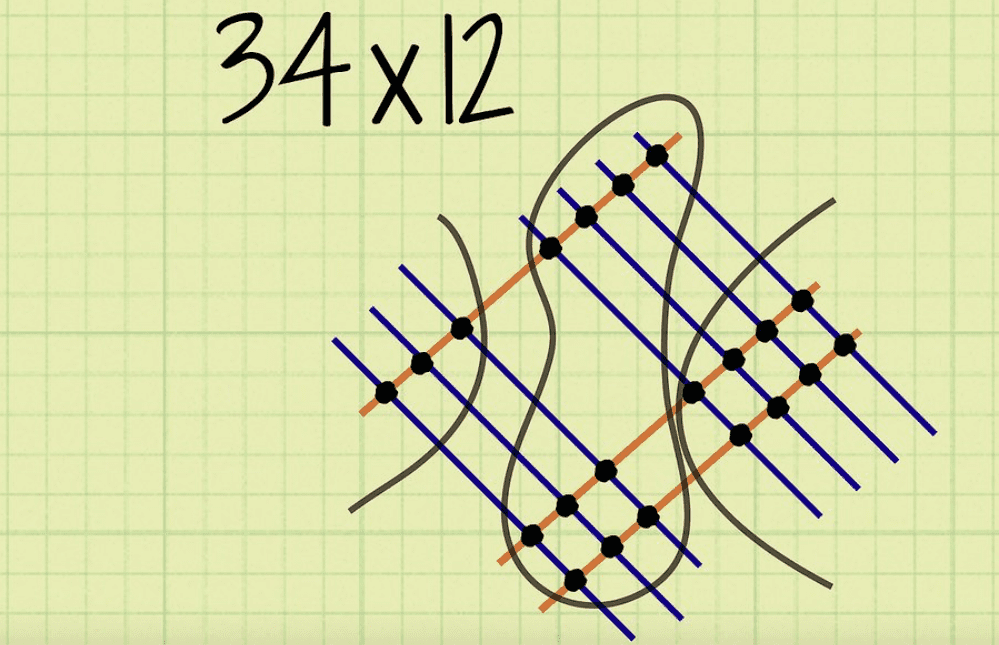Photo Credit: Wikihow

Under each circled section, add up your dots. You should count 8 for the ones and 10 for the tens.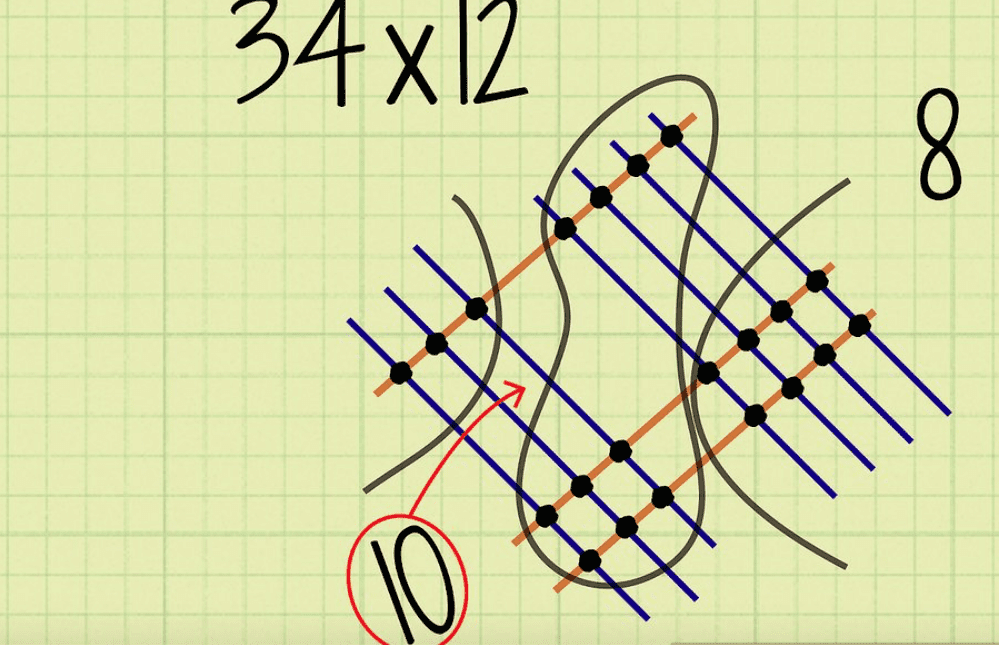Photo Credit: Wikihow

Just like in the traditional way to multiply, you will need to carry the one from the tens column over to the hundreds. Add the one to the three in the hundreds column–or the left corner. You will get four.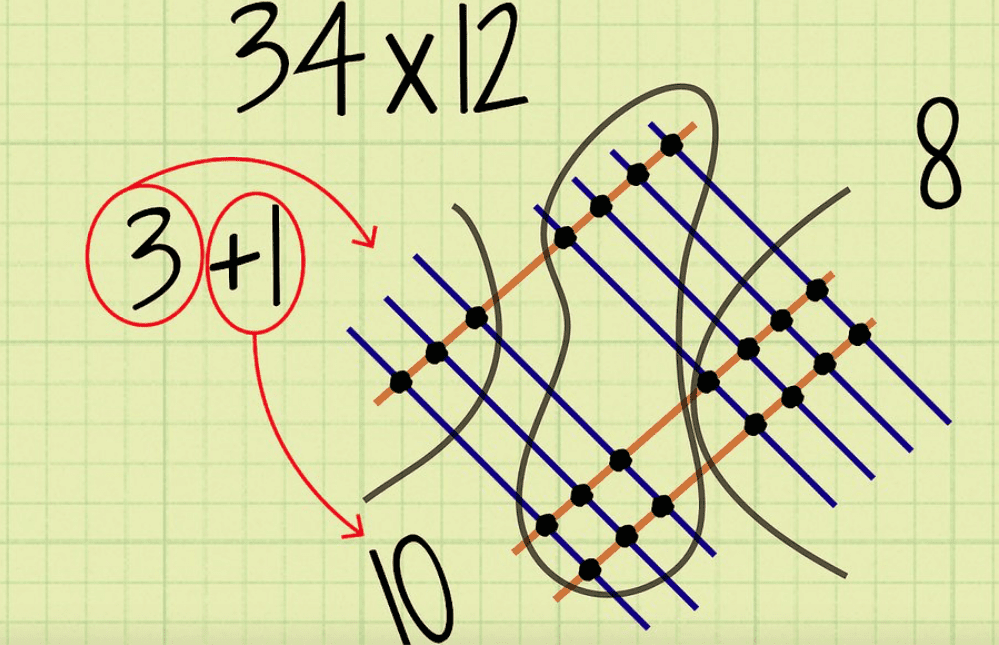Photo Credit: Wikihow

Finally, put all your digits (4 from your left corner a.k.a. the hundreds column, 0 from your middle section a.k.a. the tens column, 8 from your right corner a.k.a. the ones column) together to get your answer…

34 x 12 = 408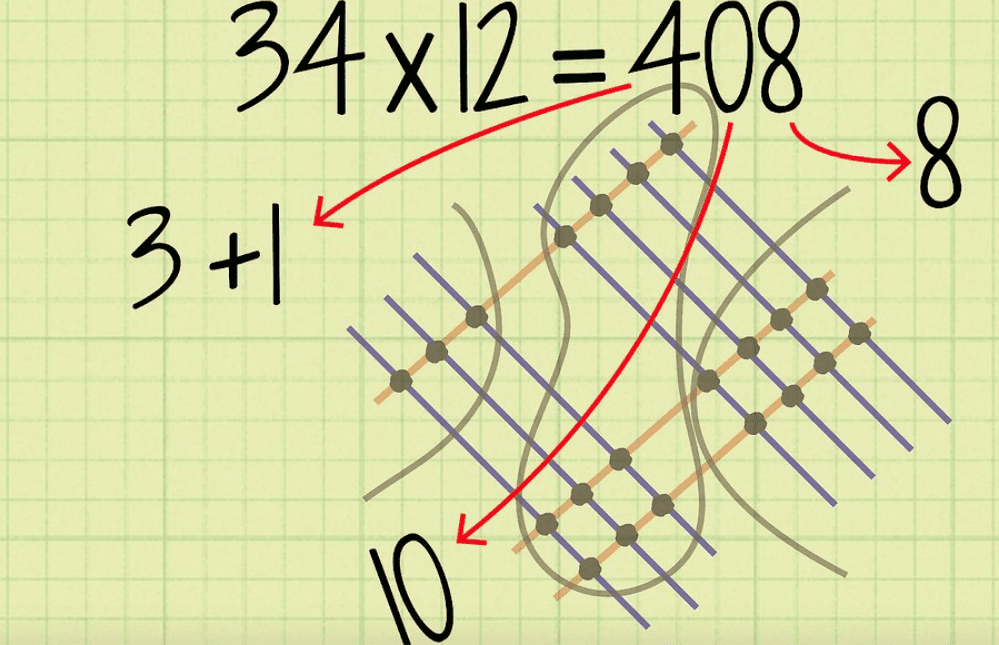Photo Credit: Wikihow

After you get the hang of it, you can use this method to multiply even larger number like triple digits. There’s more carrying over of numbers, but it’s cool to see how you can get to the right answer using this visual-based method.

If you’re a visual learner like I am, you may find this technique especially helpful.